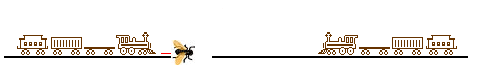The Classic "Two Trains and a Fly" problem

Changed on Wednesday, February 26, 2003 at 5:36:13 PM
Changed on Friday, January 02, 2004 - 8:46:55 PM

For another presentation, visit Dr. Math's Two Trains and a Fly.

Two trains, 20 miles apart, approach each other going 10 mph. A fly travels between them at 15 mph, zig-zagging just before getting smooshed by an oncoming train. Question: How far does the fly fly before meeting his ultimate demise?

Here's a famous story about the physicist and mathematician John von Neumann :

Another mathematician knew the quick solution to the Fly problem and wanted to see von Neumann struggle with it. He posed the question and von Neumann responded with the right answer in a few seconds.

"Interesting," said the first mathematician. "Most people try to sum the infinite series."

"What do you mean?" von Neumann replied. "That's how I did it."

Approach #1 (the "von Neumann" way)

```         --->                                        <---
<-- Dt -->                                  <-- Dt -->
.---------|---------------------------------|---------.
<-------------------  Df  ------------------>
```

(1) Let Df = the distance the fly travels on the first leg = 15*t where t = some time.
(2) Let Dt = the distance each train travels on the first leg = 10*t where t = the same time. Since
(3) Df+Dt = 20, it follows (divide (1) by (2) and use (3)) that
(4) Df/(20-Df) = 15/10. After some manipulation, this implies that
(5) Df = (3/5)*20.

It can be seen that we now have a new, similar problem. The distance has been reduced to

```     .........|---------------------------------|..........
<-----------  Df - Dt ----------->```
Using (3) and (5) it can be seen that
(6) Df-Dt = (1/5)*20.
Thus, the distance has been "shrunk" by a factor of 1/5. Applying this reasoning repeatedly, the total distance traveled by the fly is
(7) Dftotal = (3/5)*20*(1+(1/5)+(1/5)^2+(1/5)^3+ ...)
This is a "geometric series." When r<1, 1+r+r^2+r^3+ ... = 1/(1-r). (If you aren't familiar with this fact, multiply both sides by (1-r) and you'll get 1=1.) Thus,
(8) Dftotal = (3/5)*20*(1/(1-1/5)) = (3/5)*20*(5/4) = 15.

Approach #2 (the "Distance = Rate * Time" way)

It's clear it will take 1 hour before the trains crash. Therefore
(1) Dftotal = 15*1 = 15.